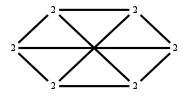The Markov Bases Database

G175_bin

The binary graphical model/graph model of K(3,3) (G175).

It is a hierarchical model of 6 variables. The dimension of the model is 15.The cardinality of the statespace is 64.

Properties of the Markov basis

 Markov degree 6 17166

 degree # of gen. 2 4 6 144 14142 2880

The model has the following properties:

• All variables are binary.
• It is a graph model and a graphical model.

The following properties of the model are unknown:

• The minimal Markov basis may be unique.FILES

 Markov basis: sufficient statistics matrix: G175_bin.mar (3.16 Mb) G175_bin.mat (4.54 kb) G175_bin.mod (71 b) G175_bin.tar.gz (121.17 kb)

Wrong or missing information? Write us an email.

page last update on November 15 2020. ->Contact